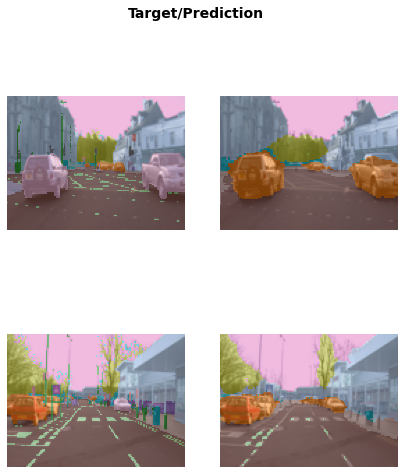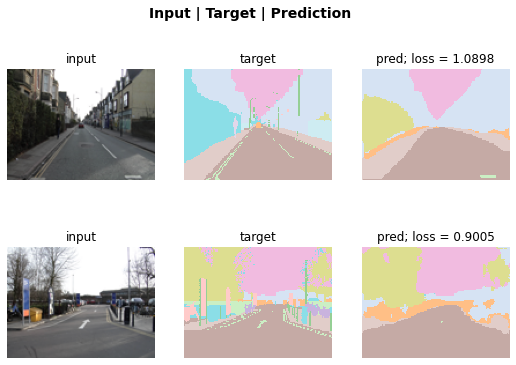# Quick start

``````from fastai.vision.all import *
from fastai.text.all import *
from fastai.collab import *
from fastai.tabular.all import *``````

fastai’s applications all use the same basic steps and code:

In this quick start, we’ll show these steps for a wide range of difference applications and datasets. As you’ll see, the code in each case is extremely similar, despite the very different models and data being used.

## Computer vision classification

The code below does the following things:

1. A dataset called the Oxford-IIIT Pet Dataset that contains 7,349 images of cats and dogs from 37 different breeds will be downloaded from the fast.ai datasets collection to the GPU server you are using, and will then be extracted.
2. A pretrained model that has already been trained on 1.3 million images, using a competition-winning model will be downloaded from the internet.
3. The pretrained model will be fine-tuned using the latest advances in transfer learning, to create a model that is specially customized for recognizing dogs and cats.

``````path = untar_data(URLs.PETS)/'images'

def is_cat(x): return x.isupper()
path, get_image_files(path), valid_pct=0.2, seed=42,
label_func=is_cat, item_tfms=Resize(224))

learn = vision_learner(dls, resnet34, metrics=error_rate)
learn.fine_tune(1)``````
epoch train_loss valid_loss error_rate time
0 0.173790 0.018827 0.005413 00:12
epoch train_loss valid_loss error_rate time
0 0.064295 0.013404 0.005413 00:14

You can do inference with your model with the `predict` method:

``````img = PILImage.create('images/cat.jpg')
img````````````is_cat,_,probs = learn.predict(img)
print(f"Is this a cat?: {is_cat}.")
print(f"Probability it's a cat: {probs.item():.6f}")``````
``````Is this a cat?: True.
Probability it's a cat: 0.999722``````

### Computer vision segmentation

Here is how we can train a segmentation model with fastai, using a subset of the Camvid dataset:

``````path = untar_data(URLs.CAMVID_TINY)
path, bs=8, fnames = get_image_files(path/"images"),
label_func = lambda o: path/'labels'/f'{o.stem}_P{o.suffix}',
)

learn = unet_learner(dls, resnet34)
learn.fine_tune(8)``````
epoch train_loss valid_loss time
0 2.882460 2.096923 00:03
epoch train_loss valid_loss time
0 1.602270 1.543582 00:02
1 1.417732 1.225782 00:02
2 1.307454 1.071090 00:02
3 1.170338 0.884501 00:02
4 1.047036 0.799820 00:02
5 0.947965 0.754801 00:02
6 0.868178 0.728161 00:02
7 0.804939 0.720942 00:02

We can visualize how well it achieved its task, by asking the model to color-code each pixel of an image.

``learn.show_results(max_n=6, figsize=(7,8))``Or we can plot the `k` instances that contributed the most to the validation loss by using the `SegmentationInterpretation` class.

``````interp = SegmentationInterpretation.from_learner(learn)
interp.plot_top_losses(k=2)``````## Natural language processing

Here is all of the code necessary to train a model that can classify the sentiment of a movie review better than anything that existed in the world just five years ago:

``````dls = TextDataLoaders.from_folder(untar_data(URLs.IMDB), valid='test')
learn = text_classifier_learner(dls, AWD_LSTM, drop_mult=0.5, metrics=accuracy)
learn.fine_tune(2, 1e-2)``````
epoch train_loss valid_loss accuracy time
0 0.594912 0.407416 0.823640 01:35
epoch train_loss valid_loss accuracy time
0 0.268259 0.316242 0.876000 03:03
1 0.184861 0.246242 0.898080 03:10
2 0.136392 0.220086 0.918200 03:16
3 0.106423 0.191092 0.931360 03:15

Predictions are done with `predict`, as for computer vision:

``learn.predict("I really liked that movie!")``
``('pos', tensor(1), tensor([0.0041, 0.9959]))``

## Tabular

Building models from plain tabular data is done using the same basic steps as the previous models. Here is the code necessary to train a model that will predict whether a person is a high-income earner, based on their socioeconomic background:

``````path = untar_data(URLs.ADULT_SAMPLE)

cat_names = ['workclass', 'education', 'marital-status', 'occupation',
'relationship', 'race'],
cont_names = ['age', 'fnlwgt', 'education-num'],
procs = [Categorify, FillMissing, Normalize])

learn = tabular_learner(dls, metrics=accuracy)
learn.fit_one_cycle(2)``````
epoch train_loss valid_loss accuracy time
0 0.372298 0.359698 0.829392 00:06
1 0.357530 0.349440 0.837377 00:06

## Recommendation systems

Recommendation systems are very important, particularly in e-commerce. Companies like Amazon and Netflix try hard to recommend products or movies that users might like. Here’s how to train a model that will predict movies people might like, based on their previous viewing habits, using the MovieLens dataset:

``````path = untar_data(URLs.ML_SAMPLE)
learn = collab_learner(dls, y_range=(0.5,5.5))
learn.fine_tune(6)``````
epoch train_loss valid_loss time
0 1.497551 1.435720 00:00
epoch train_loss valid_loss time
0 1.332337 1.351769 00:00
1 1.180177 1.046801 00:00
2 0.913091 0.799319 00:00
3 0.749806 0.731218 00:00
4 0.686577 0.715372 00:00
5 0.665683 0.713309 00:00

We can use the same `show_results` call we saw earlier to view a few examples of user and movie IDs, actual ratings, and predictions:

``learn.show_results()``
userId movieId rating rating_pred
0 5.0 3.0 2.0 3.985477
1 1.0 62.0 4.0 3.629225
2 91.0 81.0 1.0 3.476280
3 48.0 26.0 2.0 4.043919
4 75.0 54.0 3.0 4.023057
5 42.0 22.0 3.0 3.509050
6 40.0 59.0 4.0 3.686552
7 63.0 77.0 3.0 2.862713
8 32.0 61.0 4.0 4.356578